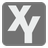Reverse Engineer
Challenging
English

You are standing next to a math machine which takes two numerical inputs x and y, and has a single numerical output. It is known that the machine evaluates an expression using x, y, the operators + – * / and brackets. You should recover the expression by using the machine and the inputs of your choice over several turns. x, y and the result you receive are integers. The machine uses real division(/) in the expression. When dividing by zero, the result will evaluate to "ZeroDivisionError".

Equivalent expressions will be accepted by the grader. For this the machine evaluates your expression and the hidden expression several times using random values.

In each step your function gets a list with data from the past steps. Each element is a list of three numbers -- x(int), y(int) and output(a fraction as a list or the string "ZeroDivisionError"). The output is represented as a list with two integers - numerator and denominator.

The function should return a list with... and , as integers.

You should be an authorized user in order to see the full description and start solving this mission.